This resource function is obsolete. Use the paclet Wolfram/QuantumFramework instead.

Function Repository Resource:

# QuantumEntangledQ

Determine whether a pair of subsystems in a discrete quantum state are entangled

Contributed by: Jonathan Gorard and Taufiq Murtadho
 ResourceFunction["QuantumEntangledQ"][QuantumDiscreteState[…],bipart] returns True if the subsystems in the bipartition bipart of the specified QuantumDiscreteState are entangled, False if they are not, or Indeterminate otherwise.

## Details

Whenever the bipartition bipart is not specified, the first and second subsystems (as specified by the bipartition {{1},{2}}) are assumed by default.
In ResourceFunction["QuantumEntangledQ"][QuantumDiscreteState[],], the specified QuantumDiscreteState should contain at least two subsystems (qudits).
The bipartition bipart should be a nested list of the general form {{i1,i2,},{j1,j2,}}, with positive integers i1,i2,,j1,j2, specifying subsystems of the given QuantumDiscreteState. For instance, the bipartition {{1,2},{3}} partitions the QuantumDiscreteState into subsystems {1,2} and {3}.
If the QuantumDiscreteState is pure, then the concurrence monotone is used to determine entanglement. If the QuantumDiscreteState is mixed, then the (logarithmic) negativity monotone is used to determine entanglement.
ResourceFunction["QuantumEntangledQ"] will try to return either True or False. However, if the concurrence and (logarithmic) negativity monotones are not defined for the specified QuantumDiscreteState and bipartition, and hence are insufficient to determine whether the specified subsystems are entangled, then ResourceFunction["QuantumEntangledQ"] will return Indeterminate.

## Examples

### Basic Examples (4)

Check whether the first and second subsystems of a two-qubit pure discrete quantum state are entangled:

 In:=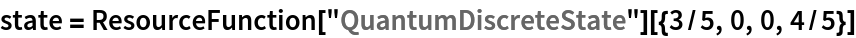Out=In:=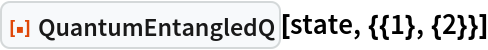Out=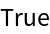Show that the three-qubit GHZ state is biseparable (i.e. any pair of qubits is separable):

 In:=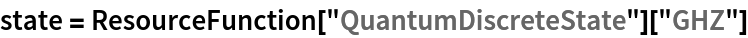Out=In:=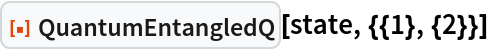Out=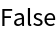In:=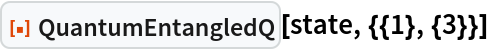Out=In:=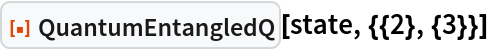Out=However, the GHZ state is not triseparable (since every triple of qubits is maximally entangled):

 In:=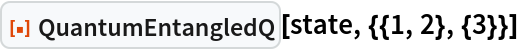Out=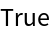Show that the three-qubit W state is not biseparable (and hence its entanglement is more robust than the GHZ state's):

 In:=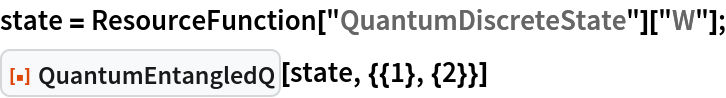Out=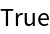Check whether subsystems 1 and 2 in a two-qubit mixed state are entangled:

 In:=Out=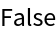Check whether subsystems 1 and 3 in a random three-qubit mixed state are entangled:

 In:=Out=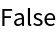Check whether subsystems {2,3} and {5} are entangled in a random 5-qubit pure state:

 In:=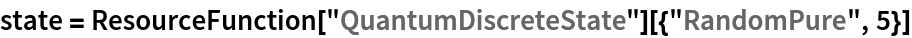Out=In:=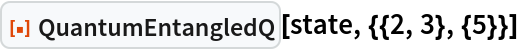Out=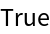### Scope (3)

QuantumEntangledQ is defined for mixed states, but only for pairs of qubits:

 In:=Out=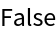For a triple of subsystems (qubits), QuantumEntangledQ is undefined (returns Indeterminate):

 In:=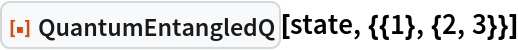Out=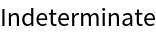Likewise, for a mixed state of qudits with dimensionality d>2, QuantumEntangledQ is undefined (returns Indeterminate):

 In:=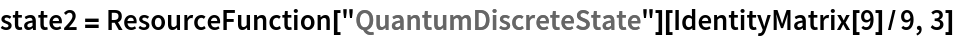Out=In:=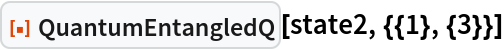Out=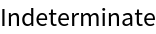Jonathan Gorard

## Version History

• 1.0.0 – 26 April 2021